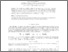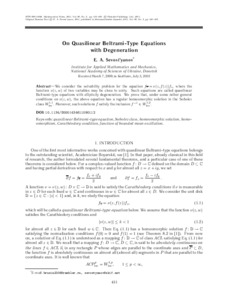# On Quasilinear Beltrami-Type Equations with Degeneration

Sevost’yanov, Е. А. (2011) On Quasilinear Beltrami-Type Equations with Degeneration. Mathematical Notes, 90 (9). pp. 431-438. ISSN 0001-4346Preview
Text

## Abstract

Abstract—We consider the solvability problem for the equation fz = ν(z, f(z))fz, where the function ν(z,w) of two variables may be close to unity. Such equations are called quasilinear Beltrami-type equations with ellipticity degeneration. We prove that, under some rather general conditions on ν(z,w), the above equation has a regular homeomorphic solution in the Sobolev classW1,1 loc . Moreover, such solutions f satisfy the inclusion f −1 ∈ W1,2 loc .

Item Type: Article quasilinear Beltrami-type equation, Sobolev class, homeomorphic solution, homeomorphism, Carathe´ odory condition, function of bounded mean oscillation. Q Science > QA Mathematics > Mathematical Analysis Faculty of Physics and Mathematics > Department of Mathematical Analysis Ірина Ігорівна Таргонська 14 Nov 2014 07:38 15 Aug 2015 10:16 http://eprints.zu.edu.ua/id/eprint/13993View Item